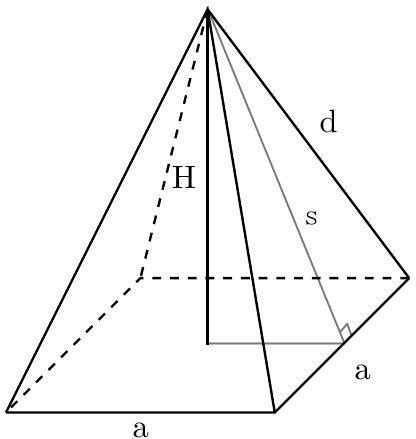# Square Pyramid Calculator

Reviewed by Purnima Singh, PhD
Last updated: Feb 02, 2023

This square pyramid calculator will help you find the total surface area and volume of any square pyramid, even if it's the famous Egyptian Pyramids (provided you know the length measurements 😉). You can also find the lateral edge of a square pyramid by using this tool. Or if you're interested in only finding the lateral surface area, this square pyramid calculator will help with that too!

## What is a square pyramid?

A pyramid, in general, has a polygonal base, with triangular lateral surfaces that meet at a point. In the case of a square pyramid, the base is surprise, surprise, a square!Here are some of the key terminologies used for square pyramids -

• The side length of the base square is called base length;
• The edges of the triangular lateral surfaces are called lateral edges of the square pyramid;
• The area of the square at the base is called the base area;
• The combined area of all the triangular lateral surfaces is called the lateral surface area of the square pyramid;
• The combined area of all 5 surfaces of the pyramid, including the base, is called the total surface area of the square pyramid; and
• The volume of the pyramid is called the square pyramid volume.

## How do I use the square pyramid calculator?

To use the square pyramid calculator for finding the surface area and volume, you may do the following -

1. Enter the square pyramid base length.
2. Enter the height of the square pyramid.
3. Tada! The square pyramid calculator will show you a host of output values, such as:
• Square pyramid base area;
• Lateral edge of the square pyramid;
• Lateral surface area of the square pyramid;
• Total surface area of the square pyramid; and
• Volume of the square pyramid.

## Other pyramid and prism calculators

The most common 3-dimensional structures we come across are pyramids and prisms. So even if what you're looking for is not the volume or surface area of a square pyramid, you can make use of some of our other calculators for other kinds of pyramid and prism computations -

## FAQ

### How many faces and edges are there in a square pyramid?

5 faces and 8 edges. Since a square pyramid has 4 lateral triangular surfaces and one square base, it has a total of 5 faces. Consequently, it has 4 lateral edges and 4 edges for the base, which add up to 8 edges in total.

### How do I find the base area of a square pyramid?

To find the base area of a square pyramid, do the following -

1. Measure the length of the base side;
2. Square that length.
3. Voila! You get the base area of the square pyramid!Base length (a)
in
Pyramid height (H)
in
Base surface area (A_b)
in²
Lateral surface parameters
Lateral edge (d)
in
Lateral surface area (A_l)
in²
Pyramid parameters
Total surface area (A)
in²
Volume (V)
cu in
People also viewed…

### Black hole collision

The Black Hole Collision Calculator lets you see the effects of a black hole collision, as well as revealing some of the mysteries of black holes, come on in and enjoy!

### Clock angle

The clock angle calculator helps you find the angle between clock hands. ⏰

### Secretary problem (Valentine's day)

Use the dating theory calculator to enhance your chances of picking the best lifetime partner.

### Standard form to general form of a circle

Standard form to general form of a circle calculator lets you convert the equation of a circle in standard form to general form.